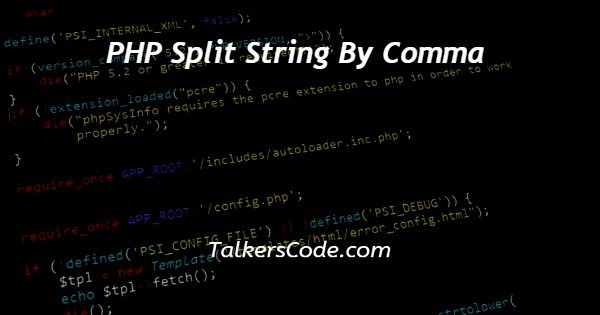# PHP Split String By Comma

Last Updated : Jan 1, 2023In this tutorial we will show you the solution of PHP split string by comma, this is mainly used to store the string in arrays in case the string in too long. In previous article, we learned about export html table data into excel.

Let us start our today’s article.

## Step By Step Guide On PHP Split String By Comma :-

Here, we are going to explain the php split string by comma and in this article, we understand about php explode () function and php preg_split() function.

Let us understand these two function first.

• explode () function: explode () function is inbuilt function of php and used to break the strings in array. This function splits the string based on string delimiter. The function returns an array containing string formed by breaking original string.
• preg_split () function: work of this function is same like explode () function, expect regular expressions are accepted as input parameters for patterns.

Now, let us understand both of them with the help of codes.

```<?php
// Use preg_split() function
\$string = "13,5050,458, 04, 099";
\$str_arre = preg_split ("/,/", \$string);
print_r(\$str_arre);
// use of explode
\$string = "0120, 87, 2, 45454, 000";
\$str_arre = explode (",", \$string);
print_r(\$str_arre);
?>
```
1. Here, first of all we already say that to run php we must knew about the basic structure of php. If you don’t about the basic structure of php then you can visit to our articles in which we learn about basic structure of php.
2. In next step, we say that we can embed html and php both in each other. There are some rules to integrate them. Please understand before working with php in html.
3. In this article, first we open and close basic tags of php. That are <?php and ?>. Now, all the material or say codes of php are written inside these codes.
4. In our example, the first code is given for preg_split() function and other is for explode () function.
5. In first case, that is preg_split, we create a string. You can see how we create this in our codes. And stored them in a variable named string also. Then we use preg_split function and use some regular expression and apply them to \$string in which we store our main string. And at last we print that string with the help of print_r() function.
6. Now, after preg_split there is explode function used. In explode, we add some strings in \$string variable and then as same in preg_split function we apply explode function and then print the stored value of explode function through \$str_arre.
7. We hope that you understand the codes properly with the help of steps given above.

## Conclusion :-

At last in conclusion, here we can say that with the help of this article we are able to understand about php split strings with the help of comma.

I hope this tutorial on PHP split string by comma helps you and the steps and method mentioned above are easy to follow and implement.